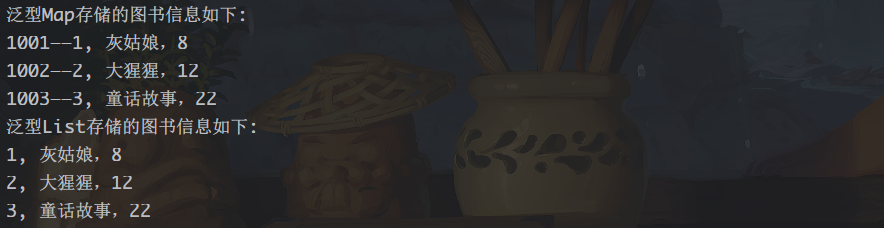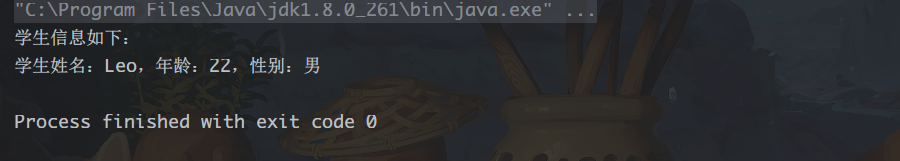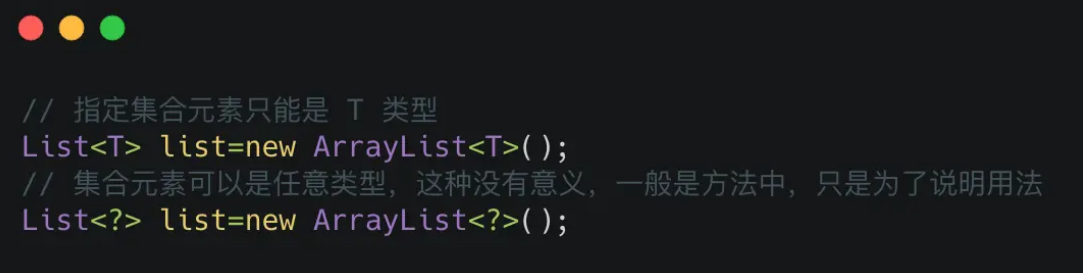## 1. 为什么会有泛型

• 适用于多种数据类型执行相同的代码

• 泛型中的类型在使用时指定，不需要强制类型转换（类型安全，编译器会检查类型

看下这个例子：

`private static int add(int a, int b) {    System.out.println(a + "+"  + b + "=" + (a + b));    return a + b;}  private static float add(float a, float b) {    System.out.println(a + "+" + b + "=" + (a + b));    return a + b;}  private static double add(double a, double b) {    System.out.println(a + "+" + b + "=" + (a + b));    return a + b;}`

`private static <T extends Number> double add(T a, T b) {    System.out.println(a + "+" + b + "=" + (a.doubleValue() + b.doubleValue()));    return a.doubleValue() + b.doubleValue();}`
`List list = new ArrayList();list.add("Leo");list.add(100d);list.add(new Person());`

`List<String> list = new ArrayList<String>();// list中只能放String, 不能放其它类型的元素`

## 2. 泛型的作用对象

### 2.1 泛型集合

#### 例 1

1）首先需要创建一个表示图书的实体类 Book，其中包括的图书信息有图书编号、图书名称和价格。Book 类的具体代码如下：

`package com.Leo.generics;/** * @author : Leo * @version 1.0 * @date 2023/8/23 22:22 * @description : Book */public class Book {    private int Id; // 图书编号    private String Name; // 图书名称    private int Price; // 图书价格    public Book(int id, String name, int price) { // 构造方法        this.Id = id;        this.Name = name;        this.Price = price;    }    public String toString() { // 重写 toString()方法        return this.Id + ", " + this.Name + "，" + this.Price;    }}`

2）使用 Book 作为类型创建 Map 和 List 两个泛型集合，然后向集合中添加图书元素，最后输出集合中的内容。具体代码如下：

`package com.Leo.generics;import java.util.ArrayList;import java.util.HashMap;import java.util.List;import java.util.Map;/** * @author : Leo * @version 1.0 * @date 2023/8/23 22:22 * @description : */public class Demo2 {    public static void main(String[] args) {        // 创建3个Book对象        Book book1 = new Book(1, "灰姑娘", 8);        Book book2 = new Book(2, "大猩猩", 12);        Book book3 = new Book(3, "童话故事", 22);        // 定义泛型 Map 集合        Map<Integer, Book> books = new HashMap<>();        // 将第一个 Book 对象存储到 Map 中        books.put(1001, book1);        // 将第二个 Book 对象存储到 Map 中        books.put(1002, book2);        // 将第三个 Book 对象存储到 Map 中        books.put(1003, book3);        System.out.println("泛型Map存储的图书信息如下:");        for (Integer id : books.keySet()) {            // 遍历键            System.out.print(id + "——");            // 不需要类型转换            System.out.println(books.get(id));        }        // 定义泛型的 List 集合        List<Book> bookList = new ArrayList<>();        bookList.add(book1);        bookList.add(book2);        bookList.add(book3);        System.out.println("泛型List存储的图书信息如下:");        for (int i = 0; i < bookList.size(); i++) {            // 这里不需要类型转换            System.out.println(bookList.get(i));        }    }}`### 2.2 泛型类

`public class class_name<data_type1,data_type2,…>{}`

`private data_type1 property_name1;private data_type2 property_name2;`

`package com.Leo.generics;public class Student<N, A, S> {    /** 姓名 */    private N name;    /** 年龄 */    private A age;    /**  性别 */    private S sex;    // 创建类的构造函数    public Student(N name, A age, S sex) {        this.name = name;        this.age = age;        this.sex = sex;    }    /** 下面是上面3个属性的setter/getter方法*/    public N getName() {        return name;    }    public void setName(N name) {        this.name = name;    }    public A getAge() {        return age;    }    public void setAge(A age) {        this.age = age;    }    public S getSex() {        return sex;    }    public void setSex(S sex) {        this.sex = sex;    }}`

`package com.Leo.generics;/** * @author : Leo * @version 1.0 * @date 2023/8/23 22:33 * @description : */public class Demo03 {    public static void main(String[] args) {        Student<String, Integer, Character> stu = new Student<>("Leo", 22, '男');        String name = stu.getName();        Integer age = stu.getAge();        Character sex = stu.getSex();        System.out.println("学生信息如下：");        System.out.println("学生姓名：" + name + "，年龄：" + age + "，性别：" + sex);    }}`1. 泛型的类型参数只能是类类型，不能是简单类型。
1. 不能对确切的泛型类型使用 instanceof 操作。如下面的操作是非法的，编译时会出错。

### 2.3 泛型方法

• 自定义的标识符(T、V、E)来代表一个类型，用`< >`括住，放在方法返回值前面。可以被用到形参声明、方法返回值、方法定义中的变量声明和类型转换。
• 泛型方法使得该泛型方法的类型参数独立于类而产生变化。泛型方法和泛型类没有关系。
• 泛型方法的类型参数，一般情况下都是被推断inference出来。更具体地讲，只能被形参或返回值推断出来，当形参和返回值用了同一个类型参数时，二者推断出来的类型必须一样、或者符合多态。
• 形参的类型参数通过实参确定；返回值的类型参数通过方法返回值赋值的对象确定。这也就是 类型参数推断
• 当形参的类型参数和返回值的类型参数是同一个时，优先使用形参的推断。因为返回值的类型参数的推断是一种拖延行为。
• 类的成员方法可以使用定义泛型类的类型参数（注意，这种方法不是泛型方法，只不过使用了类型参数而已）；而类的静态方法不可以使用泛型类的类型参数，这是因为只有当创建泛型类对象时类型参数才会被具体类型确定，也就是说，泛型类的类型参数是与对象相关的。那么，很自然地，作为一个static方法肯定不可以使用泛型类的类型参数。 static方法想用到泛型只能将其定义为泛型方法。

`[访问权限修饰符] [static] [final] <类型参数列表> 返回值类型 方法名([形式参数列表])`

`public static <T> List find(Class<T> cs,int userId){}`

`public static <T> void List(T book) { // 定义泛型方法        if (book != null) {            System.out.println(book);        }    }    public static void main(String[] args) {        Book stu = new Book(1, "Leo学Java", 28);        List(stu);        // 调用泛型方法    }`

### 2.4 泛型数组

`List<String>[] ls = new ArrayList<String>;  `

`List<?>[] ls = new ArrayList<?>;  `

`List<String>[] ls = new ArrayList;`

## 3. 泛型通配符

### 1. 常用的 T，E，K，V，？

• ？ 表示不确定的 java 类型
• T `(type)` 表示具体的一个java类型
• K V `(key value)` 分别代表java键值中的Key Value
• E `(element)` 代表Element

### 2. ？ 和 T 的区别？和 T 都表示不确定的类型，区别在于我们可以对 T 进行操作，但是对 ？ 不行，比如如下这种 ：

`// 可以T t = operate();// 不可以？ car = operate();`

T 是一个 确定的 类型，通常用于泛型类和泛型方法的定义，？是一个 不确定 的类型，通常用于泛型方法的调用代码和形参，不能用于定义类和泛型方法。